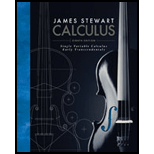# Find the number n such that ∑ i = 1 n i = 78 .### Single Variable Calculus: Early Tr...

8th Edition
James Stewart
Publisher: Cengage Learning
ISBN: 9781305270336

#### Solutions

Chapter
Section### Single Variable Calculus: Early Tr...

8th Edition
James Stewart
Publisher: Cengage Learning
ISBN: 9781305270336
Chapter E, Problem 36E
Textbook Problem
1 views

## Find the number n such that ∑ i = 1 n i = 78 .

To determine

To find: The number n such that i=1ni=78.

### Explanation of Solution

Theorem used:

Let c be a constant and n be a positive integer. Then,

i=1nc=nc, i=1ni=n(n+1)2 and i=1ni2=n(n+1)(2n+1)6.

Calculation:

By the above theorem, it is given that i=1ni=n(n+1)2.

Thus, the expression i=1ni=78 simplified as, n(n+1)2=78.

Solve the equation n(n+1)2=78 and obtain the value of n.

n(n+1)2=78n(n+1)=156n2+n156=0

The equation n2+n156=0 is a quadratic equation with variable n

### Still sussing out bartleby?

Check out a sample textbook solution.

See a sample solution

#### The Solution to Your Study Problems

Bartleby provides explanations to thousands of textbook problems written by our experts, many with advanced degrees!

Get Started

Find more solutions based on key concepts
A FALLING Stone A stone is thrown straight up from the roof of an 80-ft building, and the height (in feet) of t...

Applied Calculus for the Managerial, Life, and Social Sciences: A Brief Approach

If , then f−1(x) = x3 +3 (x + 3)3 x3 − 3

Study Guide for Stewart's Single Variable Calculus: Early Transcendentals, 8th

Describe the characteristics of a within-subjects experimental research design.

Research Methods for the Behavioral Sciences (MindTap Course List)